Courses

Shear Force & Bending Moment Diagrams Notes | Study Strength of Materials (SOM) - Mechanical Engineering

Mechanical Engineering: Shear Force & Bending Moment Diagrams Notes | Study Strength of Materials (SOM) - Mechanical Engineering

The document Shear Force & Bending Moment Diagrams Notes | Study Strength of Materials (SOM) - Mechanical Engineering is a part of the Mechanical Engineering Course Strength of Materials (SOM).
All you need of Mechanical Engineering at this link: Mechanical Engineering

A Beam is defined as a structural member subjected to transverse shear loads during its functionality. Due to those transverse shear loads, beams are subjected to variable shear force and variable bending moment.
Shear force at a cross section of beam is the sum of all the vertical forces either at the left side or at the right side of that cross section.
Bending moment at a cross section of beam is the sum of all the moments either at the left side or at the right side of that cross section.

Types of Rigid Supports

1. Simple Supports

• Roller Support
• Hinge Support (or) Pin Support

2. Fixed Supports

• Clamped Supports (or) Built-in Supports

(a) Roller Support – resists vertical forces only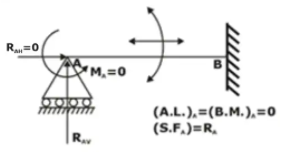(b) Hinge support or pin connection – resists horizontal and vertical forces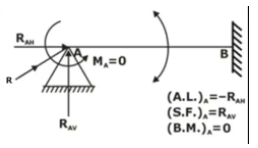(c) Fixed support or built-in end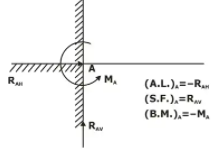Note: The distance between two supports is known as “span”.

Types of Beams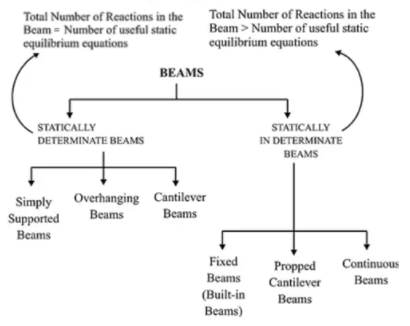1. Statically Determinate Beam: A beam is said to be statically determinate if all its reaction components can be calculated by applying three conditions of static equilibrium.
2. Statically Indeterminate Beam: When the number of unknown reaction components exceeds the static conditions of equilibrium, the beam is said to be statically indeterminate.
(i) Simply supported beam: A beam with two simple supports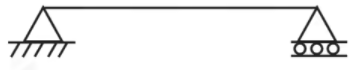(ii) Overhanging beam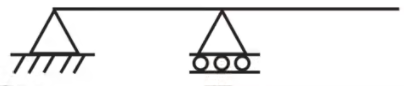(iii) Cantilever beam: Beam fixed at one end and free at other(iv) Fixed Beams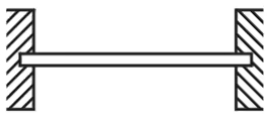(v) Propped Cantilever Beams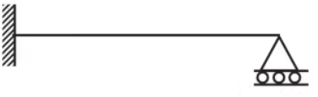(vi) Continuous beam: More than two supports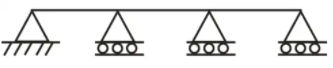The following are the important types of load acting on a beam:

•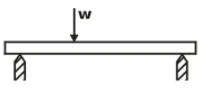Uniformly Distributed Load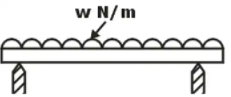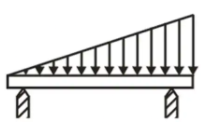Sign Conventions for Shear Force and Bending Moment

• Shear force
If moving from left to right, then take all upward forces as positive and downward as negative.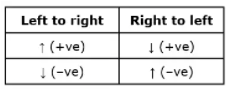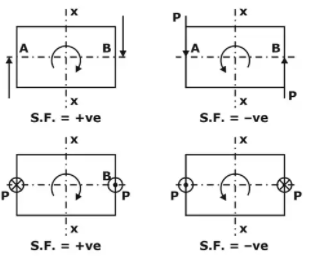Or if the shear force tries to rotate the element clockwise then it is takes as positive & if the shear force tries to rotate the element anticlockwise then it is takes as negative.

• Bending moment
If moving from left to right, take clockwise moment as positive and anticlockwise as negative.Or if forces are forming sagging moment then it is taken as positive and if forces are forming hogging moment then it is taken as negative.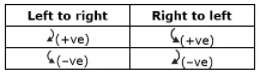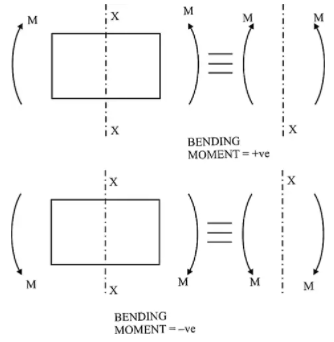Important Points for Drawing Shear Force and Bending Moment Diagrams

• Consider the left or the right portion of the section.
• The positive values of shear force and bending moments are plotted above the base line, and negative values below the base line.
• The shear force diagram will increase or decrease suddenly i.e., by a vertical straight line at a section where there is a vertical point load.
• The shear force between any two vertical loads will be constant and hence the shear force diagram between two vertical loads will be horizontal.
• The bending moment at the two supports of a simply supported beam and at the free end of a cantilever will be zero.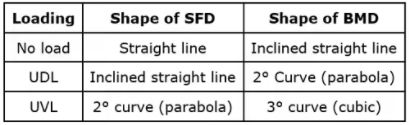Relation between Load, Shear Force and Bending Moment

A beam is carrying a uniformly distributed load of w per unit length. Consider the equilibrium of the portion of the beam between sections 1-1 and 2-2. This portion is at a distance of x from left support and is of length dx.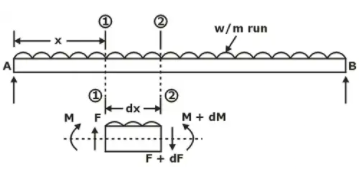F = Shear force at the section 1-1
F + dF = Shear force at the section 2-2,
M = Bending moment at the section 1-1,
M + dM = Bending moment at the section 2-2.
The forces and moments acting on the length ‘dx’ of the beam are:

• The force F acting vertically up at the section 1-1
• The force F + dF acting vertically downwards at the section 2-2.
• The load w × dx acting downwards
• The moments M and (M + dM) acting at section 1-1 and section 2-2 respectively.

The portion of the beam of length dx is in equilibrium. Hence resolving the forces acting on this part vertically, we get

–dF = w.dx

dF / dx = -w

The above equation shows that the rate of change of shear force is equal to the rate of loading.
Taking the moments of the forces and couples about the section 2-2, we get
Neglecting the higher powers of small quantities, we get
F.dx = dM

F = dM / dx
The above equation shows that the rate of change of bending moment is equal to the shear force at the section.
Some Examples: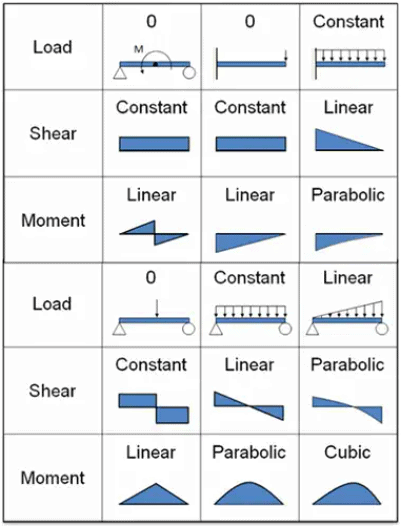The document Shear Force & Bending Moment Diagrams Notes | Study Strength of Materials (SOM) - Mechanical Engineering is a part of the Mechanical Engineering Course Strength of Materials (SOM).
All you need of Mechanical Engineering at this link: Mechanical EngineeringUse Code STAYHOME200 and get INR 200 additional OFF Use Coupon Code

Top Courses for Mechanical EngineeringStrength of Materials (SOM)

35 videos|33 docs|48 tests

Top Courses for Mechanical EngineeringTrack your progress, build streaks, highlight & save important lessons and more!

,

,

,

,

,

,

,

,

,

,

,

,

,

,

,

,

,

,

,

,

,

;